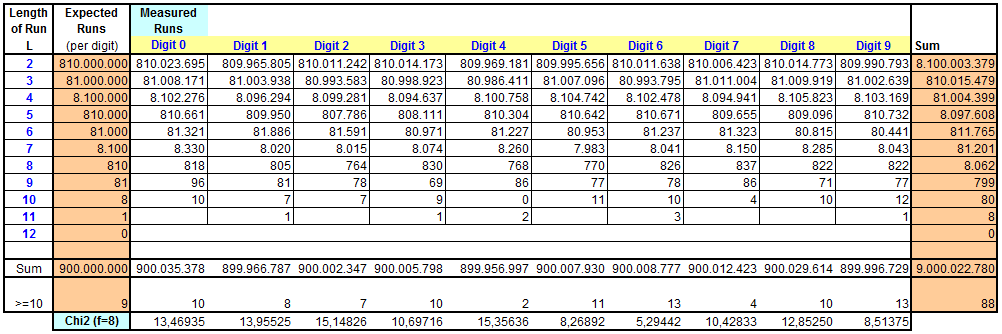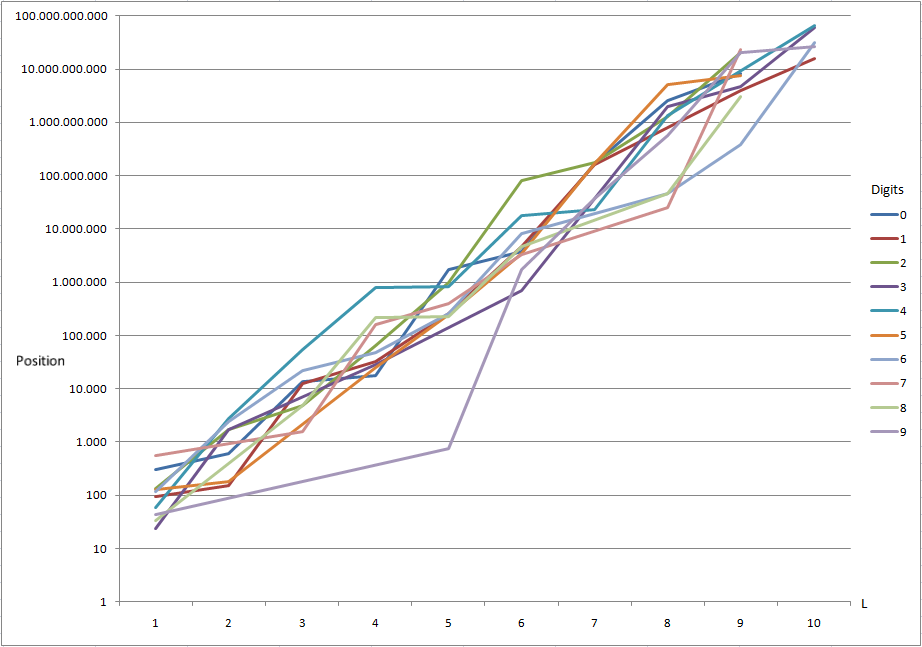# What’s the longest sequence for a certain digit?

## GENERAL CONSIDERATIONS / EXPECTED VALUES

A LONG RUN (LR) is an uninterrupted line of a certain number in a digit sequence. If the digit repeats itself M times it is also called an M-repeat. Testing LR is the opposite view to the gap test which proofs nonoccurance.
An M-repeat is a rare event because the probabilty to find exactly the same digit on the next place is 1/10 and so the chance for a LR falls down very quickly like 10-M.
When testing about 100 billion digits we will expect max long runs of 11 digits length.

A famous early LR in Pi is the FEYNMAN POINT at position 762 where a six digits repeat of "9" can be observed.

## RESULTS

Digits analyzed: 100 * 10 9
Analysis started at digit: 1

The estimated average LR-length is 1,111...
Proofing the distribution for the entire number of LR's we found a Z-value for the normal distribution  Z = 1,8532.

To calculate Chi2 values measurments for L=10 and 11 where joined in one class.
Critical Value of Chi2-Distribution on 99,5% Level (f=8): 21,955